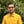Trusted answers to developer questions
Trusted Answers to Developer Questions

Related Tags

swift
atan
math
communitycreator

# What is the atan() function in Swift?Harris Amjad

Grokking Modern System Design Interview for Engineers & Managers

Ace your System Design Interview and take your career to the next level. Learn to handle the design of applications like Netflix, Quora, Facebook, Uber, and many more in a 45-min interview. Learn the RESHADED framework for architecting web-scale applications by determining requirements, constraints, and assumptions before diving into a step-by-step design process.

In Swift, the atan() function, also called the arc tangent function, is used to return the inverse tangent of a number.

Below is the mathematical representation of the atan() function:

Mathematical representation of inverse tangent function

Note: We need to import Foundation in our code to use the atan() function. We can import it like with the import Foundation command.

To convert radians to degrees, use the following formula:

degrees = radians * ( 180.0 / pi )


### Syntax

atan(number)
//number can be real, float, or double.


### Parameter

This function requires a number as a parameter.

### Return value

This function returns the inverse tangent of a number that is sent as a parameter.

The return value lies in the interval [-PI/2, PI/2] radians.

### Example

import Swiftimport Foundation//positive number in radiansprint("The value of atan(0.5) :", atan(0.5), "Radians");// negative number in radiansprint("The value of atan(-0.5) :", atan(-0.5), "Radians");//applying atan() and then converting the result in radians to degrees// radians = 1.0// PI = 3.14159265print("The value of atan(1.0): ",atan(1.0) * (180.0 / Double.pi)," Degrees");

### Explanation

• Line 2: We add the Foundation header required for the atan() function.
• Line 5: We use the atan() function to calculate the arc tangent of a positive number.
• Line 8: We use the atan() function to calculate the arc tangent of a negative number.
• Line 13: We use the atan() function to calculate the arc tangent of a positive number and convert the result to degrees.

RELATED TAGS

swift
atan
math
communitycreator

CONTRIBUTORHarris Amjad

Grokking Modern System Design Interview for Engineers & Managers

Ace your System Design Interview and take your career to the next level. Learn to handle the design of applications like Netflix, Quora, Facebook, Uber, and many more in a 45-min interview. Learn the RESHADED framework for architecting web-scale applications by determining requirements, constraints, and assumptions before diving into a step-by-step design process.

Keep Exploring

Learn in-demand tech skills in half the time